# SAT II Math I : Other 2-Dimensional Geometry

## Example Questions

### Example Question #1 : Other 2 Dimensional Geometry

Which of the following describes a triangle with sides of length 10 inches, 1 foot, and 2 feet?

This is a right triangle.

This is an acute triangle.

This is an obtuse triangle.

This triangle cannot exist.

This triangle cannot exist.

Explanation:

One foot is equal to 12 inches, so the triangle would have sides 10, 12, and 24 inches. Since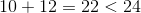,

the triangle violates the Triangle Inequality, which states that the sum of the lengths of the two smaller sides must exceed the length of the third. The triangle cannot exist.

### Example Question #2 : Other 2 Dimensional Geometry

Which of the following describes a triangle with sides of length nine yards, thirty feet, and 360 inches?

The triangle is obtuse and isosceles.

The triangle cannot exist.

The triangle is acute and isosceles.

The triangle is acute and scalene.

The triangle is obtuse and scalene.

The triangle is acute and isosceles.

Explanation:

Nine yards is equal to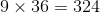inches.

30 feet is equal to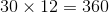inches.

In terms of inches, the triangle has sides of length 324, 360, 360; this exists since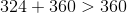and this is an isosceles triangle, since two sides have the same length.

Also,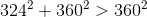,

making the triangle acute.

### Example Question #3 : Other 2 Dimensional Geometry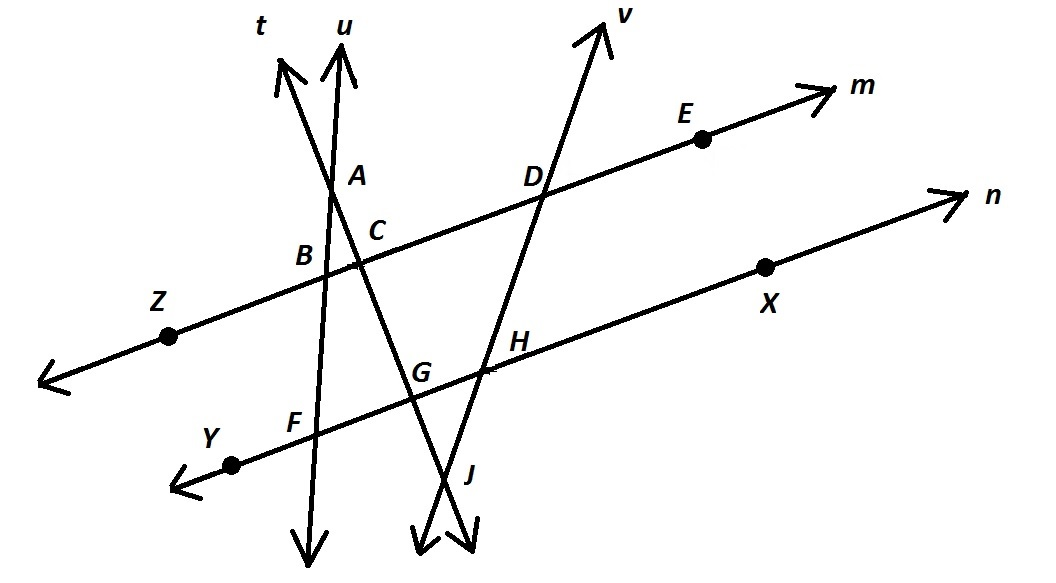Refer to the above diagram. Which of the following choices gives a set of collinear points?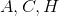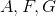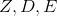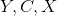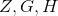Explanation:

Collinear points are points that are contained in the same line. Of the four choices, onlyfit the description, since all are on Line.

### Example Question #4 : Other 2 Dimensional Geometry

You are given triangles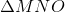and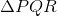, with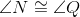. Which of these statements, along with what you are given, is enough to prove that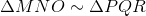?andhave the same perimeter.

None of the other responses is correct.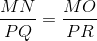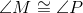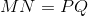Explanation:gives us the congruence of two corresponding angles and one corresponding side;  this is not enough to establish similarity.

The perimeters of the triangles are irrelevant to their similarity, soandhaving the same perimeter does not help to establish similarity, with or without what is given.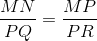establishes the proportionality of two nonincluded sides of the angles known to be congruent. However, there is no statement that establishes similarity as a result of this., along with, sets up the conditions of the Angle-Angle Similarity Postulate, which states that if two triangles have two pairs of congruent angles between them, the triangles are similar.is the correct choice.

### Example Question #5 : Other 2 Dimensional Geometry

Regular Octagon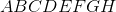has perimeter 80.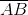hasas its midpoint; segment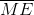is drawn. To the nearest tenth, give the length of.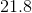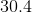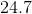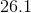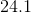Explanation:

Below is the regular Octagon, with the referenced midpointand segment. Note that perpendiculars have also been constructed fromandto meet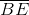atand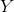, respectively.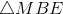is a right triangle with legs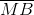andand hypotenuse.

The perimeter of the regular octagon is 80, so the length of each side is one-eighth of 80, or 10. Consequently,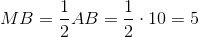To find the length of, we can break it down as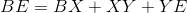Quadrilateral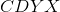is a rectangle, so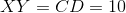.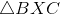is a 45-45-90 triangle with leg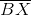and hypotenuse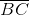; by the 45-45-90 Triangle Theorem,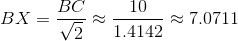For similar reasons,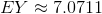.

Therefore,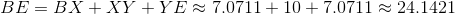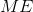can now be evaluated using the Pythagorean Theorem: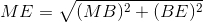Substituting and evaluating: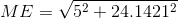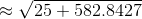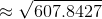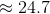,

the correct choice.